Currency Exchange Rates | IFT World
IFT Notes for Level I CFA® Program
IFT Notes for Level I CFA® Program

# Part 3

## 4. Exchange Rate Quotations

Exchange rate is the price of one currency relative to another. Exchange rates are typically quoted at four decimal places. The ratio or exchange rate is quoted as price currency per unit of base currency.

Consider this quote: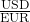= 1.4000

It means you can buy 1.4 U.S. dollars for one euro. The currency in the denominator (one unit of the currency) is the base currency. The currency in the numerator is the price currency.

The same quote may also be written as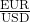= 0.7142. If you notice, it is the reciprocal of 1.4.

Direct quote: A direct quote takes domestic currency as the price currency and the foreign currency as the base currency.

Indirect quote: An indirect quote takes domestic currency as the base currency and the foreign currency as the price currency,

Direct and indirect quotes are the reciprocal of each other.

For example: From a German investor’s perspective, is= 1.4000 a direct quote?

The domestic currency for a German investor is the Euro. In this case, the Euro is shown as the base currency. Therefore, from the German investor’s perspective this quote is an indirect quote.

Bid-ask: Currencies are always quoted as bid-ask. (This is from the perspective of a dealer, not from the client’s perspective). Bid rate is the rate at which the dealer will buy the base currency. Ask rate is the rate at which the dealer will sell the base currency.

For example: A bid-ask quote of= 1.3990 – 1.4010 means that the dealer is willing to buy 1 euro for $1.399 and sell 1 euro for$1.4010.

The bid price is always lower than the sell price as the dealer makes money on the bid-ask spread.

Example

Appreciation of one currency is the depreciation of the other. Say the USD/EUR rate changed from 1.4 to 1.5. What is the appreciation/depreciation of each currency?

Solution:

The base currency is EUR; the price currency is USD.

The exchange rate goes up from 1.4 to 1.5. It means the base currency (EUR) has appreciated/strengthened. The USD has depreciated or weakened.

% appreciation of EUR =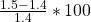= 7.142%

To calculate the depreciation in USD, we must first convert the quote in EUR/USD terms.

Take a reciprocal of the quote to get the EUR/USD values.

Initial value: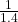= 0.7143 Later value EUR/USD =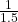= 0.6667

% Depreciation of dollar =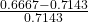= -6.67%

Note: The percentage amount by which one currency goes up (appreciates) is not necessarily the same as the percentage amount by which the other currency goes down. In our example, while the Euro appreciated by 7.142%, the U.S. dollar did not depreciate by 7.142%, instead it only depreciated by 6.67%.

## 5. Cross-rate Calculations

Given two exchange rates and three currencies, it is possible to determine the third exchange rate. This way of determining the third exchange rate by converting one foreign exchange quote into another is called the cross rate.

Given the two exchange rates below, what is the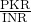rate?

 Ratio Spot rate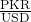100.0000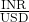60.0000

Solution: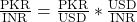In this equation, the USD cancels out giving us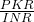.

We are given the value of. To get the value of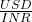, we take the reciprocal ofwhich is given.= 100 *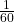= 1.667

Triangular arbitrage: If the implied cross rate is not equal to the quoted cross rate, then an arbitrage opportunity exists and it is called triangular arbitrage. In such cases, one would profit by buying low and selling high. For example, in the case above, if the bank quoted a rate of 1.8 for, then you may buy INR (sell PKR) for 1.667 and sell INR (buy PKR) for 1.8 to profit from the mispricing.

LIVE WEEKEND CLASSES *For 2024 Exam candidates*
This is default text for notification bar